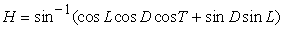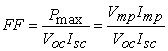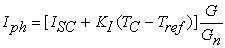﻿ Solar Power Potentiality Analysis in Some Regions of Bangladesh in the Case of Solar Irradiance

### Solar Power Potentiality Analysis in Some Regions of Bangladesh in the Case of Solar Irradiance

Suman ChowdhuryOPEN ACCESSPEER-REVIEWED

## Solar Power Potentiality Analysis in Some Regions of Bangladesh in the Case of Solar Irradiance

Suman ChowdhuryDepartment of Electrical and Electronics Engineering, IUBAT-International University of Business Agriculture and Technology

### Abstract

This paper analyzes the power characteristics of the PV cell in the case of solar irradiance. And it is also seen that a significant power change is occurred if the level of the series resistance is varied. Finally it is observed that Dinajpur is 9.67% more potential than Khulna and Chittagong in respect of solar irradiance. Also it is found that Dinajpur is 7.47% more potential than Dhaka.

### At a glance: Figures

123
Prev Next

• Chowdhury, Suman. "Solar Power Potentiality Analysis in Some Regions of Bangladesh in the Case of Solar Irradiance." Journal of Optoelectronics Engineering 2.2 (2014): 46-49.
• Chowdhury, S. (2014). Solar Power Potentiality Analysis in Some Regions of Bangladesh in the Case of Solar Irradiance. Journal of Optoelectronics Engineering, 2(2), 46-49.
• Chowdhury, Suman. "Solar Power Potentiality Analysis in Some Regions of Bangladesh in the Case of Solar Irradiance." Journal of Optoelectronics Engineering 2, no. 2 (2014): 46-49.

 Import into BibTeX Import into EndNote Import into RefMan Import into RefWorks

### 1. Introduction

Solar energy is the most promising source of clean, renewable energy and it has the greatest potential of any power source to solve the world’s energy problems [1, 2].Today more than 90% of the world market of PV cells is dominated by crystalline silicon in its multi-crystalline and mono-crystalline form. The most important things about PV cells are efficiency, long-term stability and lowest possible cost. PV cell made by mono-crystalline has been normally used because of its high efficiency. Recently, however, amorphous PV cell has its fixed place in consumer applications with the benefits of flexibility of usage and good aesthetic reason [3, 4]. After partly solving the problems of light induced degradation, amorphous silicon begins to enter the power market. The visual appearance of thin film modules makes them attractive for facade applications [5, 6].

Global radiation is the most important solar characteristics to be investigated to explore the potential of solar power generation. Global radiation in a specific location may be defined as the sum of direct and diffuse radiation throughout the day. The generated solar power is generally a global radiation on a horizontal PV panel surface. Also fixed panel receive solar radiation at angle that reduces the amount of energy considerably [7, 8]. Furthermore inclination angle and azimuth angle play a vital role in radiation surface. Azimuth angle is generated by the earth rotation with its own axis and inclination angle is produced due to rotation of the earth around the sun.

This paper tried to investigate the power performance of PV cell in the case of solar irradiance and series resistance. Realizing the importance of solar irradiance, four locations of Bangladesh are investigated for finding the solar potentiality taking solar irradiance as an active influencing factor.

The solar irradiation exposed to the PV array for fixed position is calculated by equation 1 .(1)

Where Gs is total solar irradiation in kW/m2, Gd is direct component of solar irradiation in kW/m2 and Gr is diffuse component of Solar Irradiation in kW/m2.(2)

Where H is sun elevation, θ is oblique angle of the sun in radian, α is azimuth angle,is declination of the sun in radian, φn is north latitude, ω is hour angle in degree, God is direct irradiation in radian.(3)

The sun elevation is given by the equation 4 .(4)

Where L is latitude in degree, D is declination of the sun in degree; T is hour angle in degree .

### 3. Fill Factor

Fill factor is a very important factor to measure the degree of performance. It can be defined as the closeness of the I-V curve to a square wave. Again it can be defined as the ratio of the maximum power that can be supplied to the load and product of short circuit current and open circuit voltage. The fill factor is given by (5) .(5)

Where Vmp is maximum power point voltage, Imp is maximum power point current.

Figure 1. Fill factor effect on various levels of short circuit current

From figure 1, it is seen that fill factor value is rapidly decreasing with the increment of short circuit current element taking consideration of constant maximum power and open circuit voltage.

### 4. Modeling of Pv Cell

Figure 2 shows the equivalent circuit of the PV cell. The basic equation that mathematically explains the I-V characteristics of the PV cell is shown in (6) .(6)

Where Iph is a light generated current, IS is cell saturation of dark current saturation, q (1.6×10-19C) is electron charge, k is a Boltzmann’s constant (1.38×10-23J/k), TC is the cell’s working temperature, A is an ideality factor, Rsh is a shunt resistance and RS is a series resistance. The light generated current mainly depends on the solar irradiance and cell’s working temperature which is described by mathematical expression shown by (7) .(7)

Where ISC is the cell’s short circuit current at 25°C, KI is the cell’s short circuit current temperature coefficient, Tref is the cell’s reference temperature and G is the solar irradiation in kW/m2 and Gn is nominal solar irradiation at STC (Standard test condition) in kW/m2. Again the cell’s saturation current varies with cell temperature which is expressed in (8) .(8)

Where IRS is the cell’s reverse saturation current at reference temperature, EG is the band-gap energy of semiconductor material utilized in the PV cell.

The cell’s reverse saturation current which is related to open circuit voltage and short circuit current is given by (9) .(9)

Where ΔT is the temperature difference between the actual and nominal temperature (°C), VOC is the open circuit voltage of the PV cell, KV is voltage coefficient and Vt is the thermal voltage of PV cell. The thermal voltage is given by (10).(10)

### 5. Modeling of PV Array

The PV array is the series or parallel combination of the modules. To obtain power at desired voltage and current, the series and parallel combination of the PV modules should be analyzed properly. The current and voltage relationship of the PV array having NS series and NP parallel is given by (11) .(11)

### 6. Simulation of the PV CELL and Solar Irradiance Data Analysis

To simulate the PV cell a solar model is taken known as BP MSX 64. The short circuit current and open circuit voltage of the MSX 64 solar module is 4A and 21.3V respectively. The basic simulink model for the PV cell is shown in Figure 3. Figure 4 shows the significant effect of solar irradiance on power of solar cell. From figure, it is spontaneously clear that power level is rising up significantly with the increment of incident solar irradiance. And from Figure 5, it is seen that maximum power is obtained when the series resistance value is minimum. So it can be said that solar power could be optimum if the least value of series resistance is maintained.

In addition to this, solar irradiance data has been analyzed for some particular locations in Bangladesh. The data has been taken from NASA website. Figure 6, Figure 7, Figure 8 and Figure 9 represent monthly averaged (22 year data) solar irradiance data for Khulna, Chittagong, Dhaka and Dinajpur respectively. The maximum solar irradiance observed is 5.83 KWh/m2-day in Khulna region whether it is 5.51, 5.76 and 6.47 KWh/m2-day in Chittagong, Dhaka and Dinajpur respectively in April. And the minimum solar irradiances observed are 3.83, 4.01, 4.01 and 3.99KWh/m2-day in Khulna, Chittagong, Dhaka and Dinajpur respectively in September month.

### 7. Conclusion

The power performance of the PV cell has been analyzed using the simulink of MATLAB software. The influencing factors considered are solar irradiance and series resistance. For the significant of the solar irradiance on solar power of PV cell, solar irradiance data has been analyzed in some particular regions of Bangladesh. From the respect of solar irradiance, it is found that Dinajpur is more potential than the other three regions. From the solar irradiance data, it is seen that maximum annual averaged solar irradiance is occurred in Dinajpur region which is around 4.99 KWh/m2-day.

### References

  M. Z. A. A. Kadir and Y. A. Rafeeu, “A review on factors for maximizing solar fraction under wet climate environment in Malaysia”, Renew. Sust. Ener. Rev., 2010, 14, 2243-2248.In article CrossRef  A. O. Adelaja, O. Damisa, S. A. Oke, A. B. Ayoola and A. O. Ayeyemitan, “A survey on the energy consumption and demand in a tertiary institution”, Maejo Int. J. Sci. Technol., 2008, 2, 331-344.In article  Maurus H, Schmid M, Blersch B, Lechner P, Schade H: PV FOR BUILDINGS: Benefits and experiences with amorphous silicon in BIPV applications: reFOCUS 2004; 5 (6): 22-27.In article CrossRef  Wong PW, Shimoda Y, Nonaka M, Inoue M, Mizuno M: Semi-transparent PV: Thermal performance, power generation, daylight modeling and energy saving potential in a residential application: Renewable Energy 2008; 33 (5): 1024-1036.In article CrossRef  Goetzberger A, Hebling C, Schock HW: Photovoltaic materials, history, status and outlook: Materials Science and Engineering 2003; 40 (1): 1-46.In article CrossRef  Muller A, Ghosh M, Sonnenschein R, Woditsch P: Silicon for photovoltaic applications: Materials Science and Engineering: B 2006; 134 (2-3): 257-262.In article CrossRef  Luque, A., Hegedus, S.: Handbook of Photovoltaic Science and Engineering. John Willey & Sons Ltd., 2003.In article CrossRef  Hanitsch, R., Etier, N., Heumann, K., Munschauer, M., Simulation and comparison of a tracked PV system with a model based on the measurement of the sky irradiance distribution, EuroSun98, 2nd ISES-Europe, Portoroz, Slovenia, 1998.In article  R. Muhida, M. Ali, P. S. J. Kassim, M. A. Eusuf, A.G.E. Sutjipto, and Afzeri, “A simulation method to find the optimal design of photovoltaic home system in Malaysia”, case study: a building integrated photovoltaic in Putra Jaya.In article  S. Thanakodi, “Modeling and simulation of grid connected photovoltaic system using MATLAB / SIMULINK”, Malaysia Nov.-2009.In article  H. L. Tsai, C. S. Tu, and Y.J. Su, “Development of generalized photovoltaic model using MATLAB/ SIMULINK” Proceedings of the World Congress on Engineering and Computer Science 2008, October 22-24, San Francisco, USA.In article  M. G. Villalva, J. R. Gazoli and E. R. Filho, “Comprehensive approach to modeling & simulation of photovoltaic arrays”, IEEE Transactions on Power Electronics, vol. 24, no. 5, May 2009.In article CrossRef  J. Durago, “Photovoltaic emulator adaptable to irradiance, temperature and panel-specific I-V curves”, California polytechnic state university. June 2011.In article